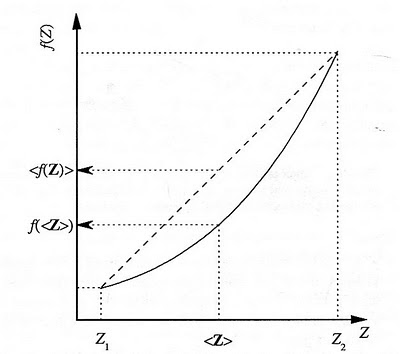## Friday, November 12, 2010

### Jensen's Inequality

Jensen's inequality is a simple math relationship that everyone should know. Like most mathematical relationships, it can be written with enough symbols to make it impossible to understand, but the qualitative idea is very simple: if you have a nonlinear relationship between an input and an output, and if you look at the output from those two inputs, the average of the outputs is not equal to what you would find for the output from the average of the inputs. Too wordy, I know. Let me give you an example.

This picture below is a good graphic of the situation.The two inputs are Z1 and Z2, and you can also see the corresponding values f(Z1) and f(Z2) which unfortunately are not labeled. Halfway between Z1 and Z2 is their average, < Z > and halfway between f(Z1) and f(Z2) is < f(Z) >, which you can see is greater than f(< Z >). So this then is Jensen's inequality:
< f(Z) > ≥ f(< Z >)
In this case (and in the example above), the nonlinear relationship is concave up, so the "average" output is greater than the output of the average input. Certainly concave down relationships can happen, and the outcome will be the opposite.

Chemical reactions have a non-linear relationship with temperature (i.e., the Arrhenius equation). So if you first run a reaction for a certain amount of time at one temperature, T1, and then change the temperature to T2 and run it for the same amount of time, the extent of the reaction will be greater than if you had run it at the average temperature, (T1 + T2)/2. Why? Given the non-linear nature of the reaction to temperature, the reaction runs quite a bit faster at the higher temperature compared to how much the colder temperature slows it down.

I've used this relationship in the past quite a bit when I was working with perishable food items. The food was never stored at a constant temperature - even properly working refrigerators cycle around a set point, so we used data loggers to record the temperature at regular intervals. The software that came with the data loggers actually would calculate the "average" temperature, a value that you can see is meaningless. Instead we would calculate a "mean kinetic temperature". Using the Arrhenius equation, we would determine the extent of degradation during each time interval, sum up the total extent of the reaction, and then back calculate the temperature that would have given us that reaction extent. (This is trivial to set up in a spreadsheet - import the data, hit F9 and you're done.) The mean kinetic temperature was always higher, and the higher the activation for the food, the greater the deviation.[*]

I was directly reminded of the inequality today when I came across a paper that looked at the implications of Jensen's inequality as applied to Ca2+ oscillations in cells. Surprisingly, the cells do much better than when the output is steady, and this was shown to be a direct result of the inequality.

[*] This is what I was getting at a few weeks ago after the power went out and we had to triage the food in the fridge. I tossed all the food with high activation energies since Jensen's inequality greatly disfavored them after temperature abuse.Anonymous said...

Good explanation, thanks!Anonymous said...

Thanks, John. This is the best explanation in the internet. Congratulations.Anonymous said...

you are a genius man .... thanka a lot !!